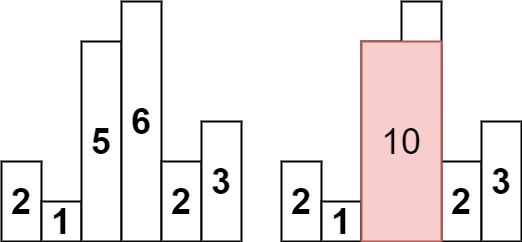# Leetcode 84 - Largest rectangle in histogram

Note:

• For each col, we need to find the first col that is shorter than it on both left and right.
• Use brute force will give us a O(n^2) algorithm. Can we finish it in just one iteration?
• Is there a data structure that can quickly give us the first col that is shorter than the current col on the left and right?
• Monotonic queue!
• For an element stack[i] in stack, stack[i-1] is the first element shorter than stack[i] on the left.
• What about right side? When we got a real shorter col and need to pop many cols including stack[i], we found the first shorter col on the right.
• Use monoStack[] to store indexes of cols.
• How to calculate area of a rectangle?
•• For example, the current stack[] is [1,4,7], which is corresponding to heights like [2,5,8], the next col height is 6.
• How do we know the WIDTH of col with height 8?
• Pop up the top of stack first as top.
• Clearly, the width is between cols with height 5 and 6.
• Right now the stack is like [1, 4].
• The width = i - stack[stack.length - 1] - 1, which is 3 (index 5, 6, 7).
• Area = height[top] * width.
• DETAILS:
1. What if the cols are strictly increasing? We don’t even have a chance to calculate the area.
• Add 0 to the end of heights[]!!
• In this way, we can ensure that we’ve pop out all cols after all iterations.
1. When there is only one element left in the stack, there is stack[stack.length - 1] is UNDEFINED!
• Add 0 to the start of heights[] as well!!

Question:

Given an array of integers heights representing the histogram’s bar height where the width of each bar is 1, return the area of the largest rectangle in the histogram.

Example:Code: